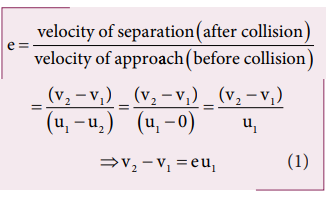Home | | Physics 11th std | Coefficient of restitution (e)

# Coefficient of restitution (e)

The rubber ball will bounce back higher than the plastic ball.

Coefficient of restitution (e)

Suppose we drop a rubber ball and a plastic ball on the same floor. The rubber ball will bounce back higher than the plastic ball. This is because the loss of kinetic energy for an elastic ball is much lesser than the loss of kinetic energy for a plastic ball. The amount of kinetic energy after the collision of two bodies, in general, can be measured through a dimensionless number called the coefficient of restitution (COR).

It is defined as the ratio of velocity of separation (relative velocity) after collision to the velocity of approach (relative velocity) before collision, i.e.,In an elastic collision, we have obtained the velocity of separation is equal to the velocity of approach i.e.,This implies that, coefficient of restitution for an elastic collision, e =1. Physically, it means that there is no loss of kinetic energy after the collision. So, the body bounces back with the same kinetic energy which is usually called as perfect elastic.

In any real collision problems, there will be some losses in kinetic energy due to collision, which means e is not always equal to unity. If the ball is perfectly plastic, it will never bounce back and therefore their separation of velocity is zero after the collision. Hence, the value of coefficient of restitution, e = 0.

In general, the coefficient of restitution for a material lies between 0 < e <1.

## Solved Example Problems for Coefficient of restitution (e)

### Example 4.22

Show that the ratio of velocities of equal masses in an inelastic collision when one of the masses is stationary is

v1/v2 = 1-e/1+e

### SolutionFrom the law of conservation of linear momentum,Using the equation (2) for u1 in (1), we getStudy Material, Lecturing Notes, Assignment, Reference, Wiki description explanation, brief detail
11th Physics : UNIT 4 : Work, Energy and Power : Coefficient of restitution (e) |